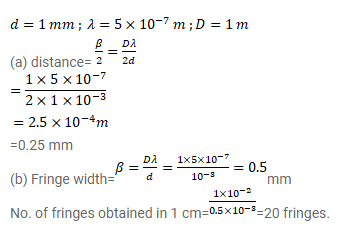# In a double slit interference experiment,Question:

In a double slit interference experiment, the separation between the slits is $1.0 \mathrm{~mm}$, the wavelength of light used is $5.0 \times 10^{-7} \mathrm{~m}$ and the distance of the screen from the slits is $1.0 \mathrm{~m}$.

(a) Find the distance of the centre of the central maximum.

(b) How many bright fringes are formed in one centimeter width on the screen?

Solution: